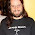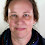## Pic## Monday, November 11, 2013

### Exponents Without Repeated Multiplication

The Problem With Exponents Education

Kids have trouble learning exponents. In 5th and 6th Grade they regularly multiply the base and the power instead of performing exponentiation. In 9th Grade they think that negative exponents must yield negative results, and in 11th Grade they struggle to figure out what in the world a fractional power might mean. And, if you push them hard enough, they'll often reveal a tendency to still multiply the base and the power.

What is the source of all these troubles? One answer is that exponents are introduced to kids solely as repeated multiplication

You might not find this troubling. You might say, "Hey, that's a fine mathematical definition. After all, isn't multiplication just repeated addition?"

In math we deal in abstractions, and abstractions are best understood from multiple perspectives. Sometimes it's helpful to think of multiplication as repeated addition. But is 3.4 times 5.7 really best understood as repeated addition of 5.7 some 3.4 times? Instead, you might think that there are times when area is a good model for multiplication. Other times call for scaling as our model. Arrays are often helpful. The number line is often useful.

The point is that, in multiplication, we have multiple models that help us glimpse aspects of what is essentially an abstraction. For thinking about the Distributive Property, you want the array model. But arrays are less helpful for decimal multiplication, and there you really want to be talking about area with your students. For multiplication of negative numbers, you want transformations of the number line. Different models for different moments.

We have one way of thinking about exponents, and it's not terribly effective all on its own. We need more models for exponentiation, and we need to think about replacing "repeated multiplication" as our students' first exponents model.

In the early grades, students should be able to talk (talk!) about squaring and cubing a length, and that's it. You don't worry about the notation. You don't talk about anything besides lengths. You don't talk about powers. You don't do anything besides squaring and cubing lengths, and getting kids used to that language.

You might kick that unit off with a visual pattern such as this one:

You make sure that kids can draw the next step. You ask them what the 7th picture looks like, and you ask them how many bricks are in that picture. You use these problems to give them practice with multiplication and you use the language of "squared" persistently.

Then you toss this in front of them:

4th Graders will find these problems much more challenging. They'll have trouble drawing 3D models, and you should teach them to sketch drawings of cubes, an important Geometric skill that's often never taught to kids explicitly. And, though you start by asking them how many cubes are in the fourth picture, pretty soon you're asking them "What is 4 cubed?"

And, if your kids are anything like mine, they will absolutely not figure this out by multiplying 4 by 4 by 4. Instead they'll start trying to calculate 16 times 4.

This difference is subtle enough that you might dismiss it, but you shouldn't. 16 times 4 is an entirely different conceptual model for cubing than 4 times 4 times 4 is. You and your class should make the relationship between "Something cubed" and "That same thing squared" explicit by the end of your study.

This, and nothing else, should be kids' first exposure to exponents.

The Next Models

Of course the Geometric model of exponentiation will hardly suffice for kids in the long run. But it's the foundation, it's where we start. And what we're going to do for kids is extend the operation of squaring and cubing to other powers,to fourth and fifth and seventh powers. To do this, we'll need another model for exponentiation.

For extension to other powers we'll rely heavily on Geometric series, our second model of exponentiation. If we want to, we can even be fancy and explicitly connect the old model with our new one:

And, again, the spoken language that we use is pretty much the point here. We're going to start talking about the picture after the cube as the "Fourth Power of Three." More generally, we're going to talk about powers as entries in these patterns.

What's going on here is that we're moving toward a third model, which is a recursive definition of exponents, with squaring and cubing as our anchors. We'll define "3 to the fourth power" for students as multiplying "3 cubed" by 3. After all, isn't that how we moved from 3 squared to 3 cubed back in our earlier work?

Still not convinced that the recursive model is a worthwhile investment? Think ahead to how hard it is to convince kids that negative exponentiation doesn't have to produce negative results, and think about how helpful these patterns will be for that.

Of course, now that you have extended exponents beyond squaring and cubing, you might find it interesting to revisit that earlier model with your kids. Can we make sense of raising a length to the first power? Can we make sense of raising a length to the fourth power?

We have three models -- Geometric, Geometric Series, and Recursive Definition -- and we haven't said a word about repeated multiplication. So where is repeated multiplication in all this? It's festering in the classroom. Some kids have figured out that you can skip steps in the recursive definition using repeated multiplication. Kids have shared this as one of a few computation strategies for doing exponents calculations. (Others are skip counting to follow the Geometric Series or using exponent properties to take shortcuts, e.g. 3^5 is 9 x 27.)

And, then? Then you make it explicit. You talk about the repeated multiplication definition, because that's a really important model for exponentiation also. In particular, it's one way to see that negative exponents often create fractional values. (Though, as evidenced by student errors, it's not a particularly effective tool on its own.) Seeing exponentiation as repeated multiplication makes simplifying expressions easier. (But, again, this is an area that is currently riddled with student errors.) So we should teach repeated multiplication to kids explicitly, and this should happen some time in (how about) 6th Grade.

But the foundations for exponentiation need to be laid several years earlier in a child's education if we want to really help our kids avoid all those pesky, Algebra-killing errors in high school.

1.Great ideas about how to teach exponents, and how to start getting them in earlier.

I think you have a typo in one of your images: it goes 1, square, cube instead of 1, line, square, cube. And probably the 1 should be a point?

1.Bah, you're right. I'll fix this and update the post.

2.Michael
Lovely stuff here. I shared it out to my Middle School team and my Alg I and Alg II teachers. Let's start a revolution here!

1.Can it be a bloody one?

3.I think I've pointed here before, but now I get to do it with a new question: http://christopherdanielson.wordpress.com/2013/04/11/rational-exponents-third-grade-style/ -- in this story, is Griffy using the recursive definition?

I wonder if doubling is special in the minds of children (i.e. easier to access) as compared to tripling, quadrupling, etc.

I wish I had some 3rd and 4th graders to hang out with this year...

1.Er, I meant Recursive Model not Recursive Definition.

2.Thanks for this, Max. I should say, this post is pretty explicitly intended as a response to Danielson's. It's his suggestion that we look for something beyond repeated multiplication that I'm really running with here.

I think that doubling isn't a great model for exponents. First, because we have a fairly limited vocabulary in English for things of that sort. We have doubling, tripling, quadrupling, and then things get kind of hairy. I think that we're going to want to be able to talk about powers of all sorts of numbers, and not want to limit ourselves to just a few special cases.

I'd say that Griffy isn't using the recursive model because there's no connection here to anything having to do with powers. Kids can follow doubling patterns with relative ease. The trick is to use that to build a network of knowledge about powers.

Or, maybe I'm still sort of confused.

4.Have you taken a look at Vi Hart's latest video on logarithms? It does a good job (IMHO) of laying out the relationship of fractional exponentiation. (And I think it would be fairly straightforward to extend the ideas to negative exponents, simply by adapting the existing knowledge about negative numbers on the number line into the log-space).

5.Don't give up on the geometric model too fast! It is possible to continue without going into the 4th dimension, and in fact it solves one problem that I had with the fractal model. You've already shown that:
3^0=single block=1
3^1=line of 3 blocks=3
3^2= square=9
3^3=cube=27
Now look at the cube as a new "single" block:
3^4=row of cubes=81
3^5=square of cubes=243
3^6=cube of cubes, which becomes the new unit for the next steps:
3^7=row of cube of cubes, etc.

The problem this solves (for me) is that it's very easy to think of negative exponents as cutting the block into pieces, so we have an intuitive way of understanding negative exponents. With the fractal model, I didn't see an intuitive way to continue the 5-pattern into fifths and fifths of fifths. But with the geometric model, it's easy to imagine continuing the pattern: slicing a single block into square thirds, and then slicing that into row-like thirds of thirds, and then cutting that into block-like thirds of thirds of thirds, and so on forever.

I'm still waiting for a good way to help my students make intuitive sense of fractional exponents.

6.This and Snap Hotel have definitely inspired me to invest in unifix cubes for the middle school classroom! Thanks.